# TensorFlow 数据集和估算器介绍

TensorFlow 1.3 引入了两个重要功能，您应当尝试一下：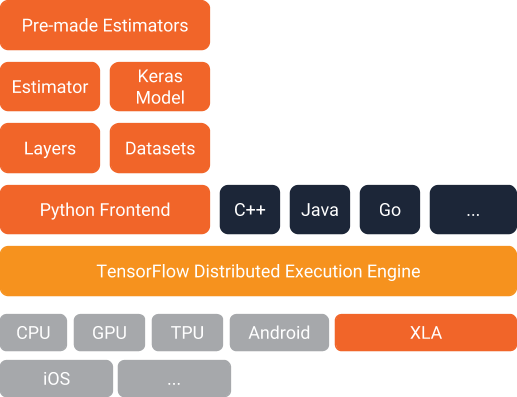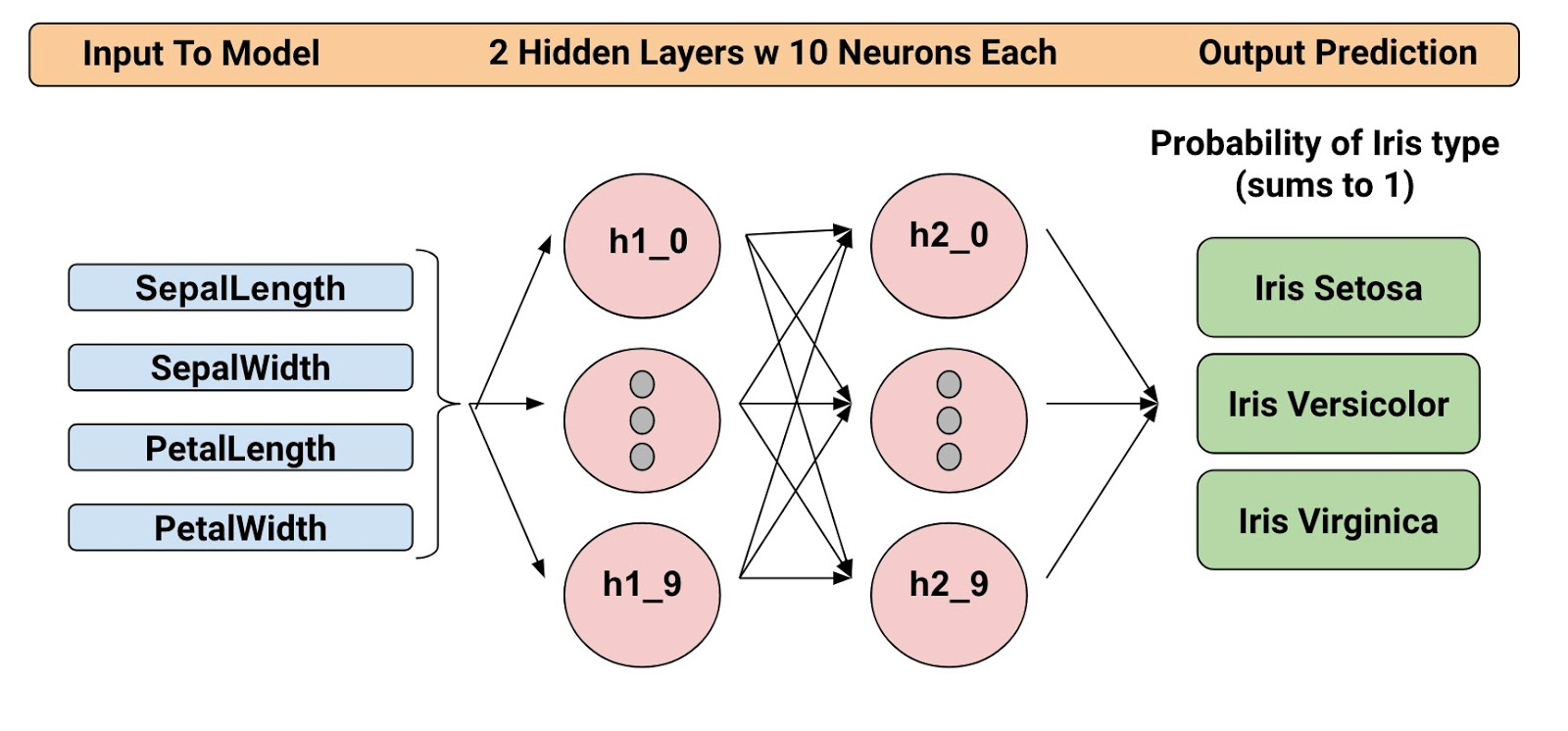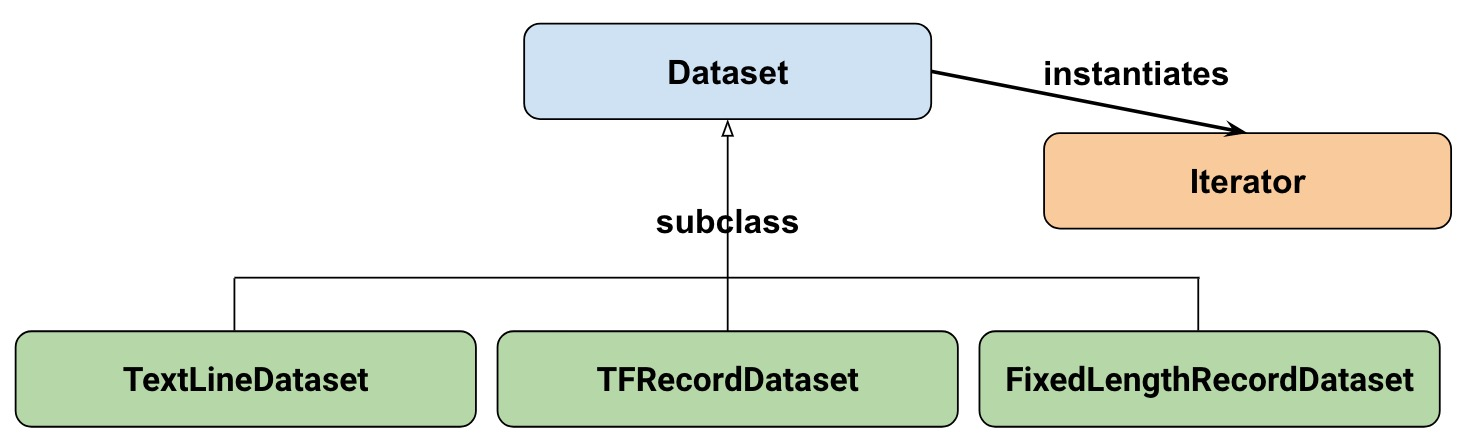TextLineDataset：从文本文件中读取各行内容。
TFRecordDataset：从 TFRecord 文件中读取记录。
FixedLengthRecordDataset：从二进制文件中读取固定大小的记录。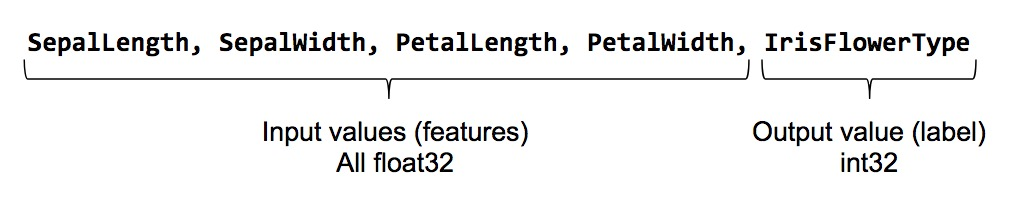[code lang=text]
feature_names = [
'SepalLength',
'SepalWidth',
'PetalLength',
'PetalWidth']

[/code]

[code lang=text]
<br />def input_fn():
…<code>…
return ({ 'SepalLength':[values], ..<etc>.., 'PetalWidth':[values] },
[IrisFlowerType])
[/code]

file_path：要读取的数据文件。
perform_shuffle：是否应将记录顺序随机化。
repeat_count：在数据集中迭代记录的次数。例如，如果我们指定 1，那么每个记录都将读取一次。如果我们不指定，迭代将永远持续下去。

[code lang=text]
def my_input_fn(file_path, perform_shuffle=False, repeat_count=1):
def decode_csv(line):
parsed_line = tf.decode_csv(line, [[0.], [0.], [0.], [0.], ])
label = parsed_line[-1:] # Last element is the label
del parsed_line[-1] # Delete last element
features = parsed_line # Everything (but last element) are the features
d = dict(zip(feature_names, features)), label
return d

dataset = (tf.contrib.data.TextLineDataset(file_path) # Read text file
.map(decode_csv)) # Transform each elem by applying decode_csv fn
if perform_shuffle:
# Randomizes input using a window of 256 elements (read into memory)
dataset = dataset.shuffle(buffer_size=256)
dataset = dataset.repeat(repeat_count) # Repeats dataset this # times
dataset = dataset.batch(32) # Batch size to use
iterator = dataset.make_one_shot_iterator()
batch_features, batch_labels = iterator.get_next()
return batch_features, batch_labels

[/code]

TextLineDataset：在您使用 Dataset API 的文件式数据集时，它将为您执行大量的内存管理工作。例如，您可以读入比内存大得多的数据集文件，或者以参数形式指定列表，读入多个文件。
shuffle：读取 buffer_size 记录，然后打乱（随机化）它们的顺序。
map：调用 decode_csv 函数，并将数据集中的每个元素作为一个参数（由于我们使用的是 TextLineDataset，每个元素都将是一行 CSV 文本）。然后，我们将向每一行应用 decode_csv 。
decode_csv：将每一行拆分成各个字段，根据需要提供默认值。然后，返回一个包含字段键和字段值的字典。map 函数将使用字典更新数据集中的每个元素（行）。

[code lang=text]
next_batch = my_input_fn(FILE, True) # Will return 32 random elements

# Now let's try it out, retrieving and printing one batch of data.
# Although this code looks strange, you don't need to understand
# the details.
with tf.Session() as sess:
first_batch = sess.run(next_batch)
print(first_batch)

# Output
({'SepalLength': array([ 5.4000001, …<repeat to 32 elems>], dtype=float32),
'PetalWidth': array([ 0.40000001, …<repeat to 32 elems>], dtype=float32),

},
[array([, …<repeat to 32 elems>], dtype=int32) # Labels
)

[/code]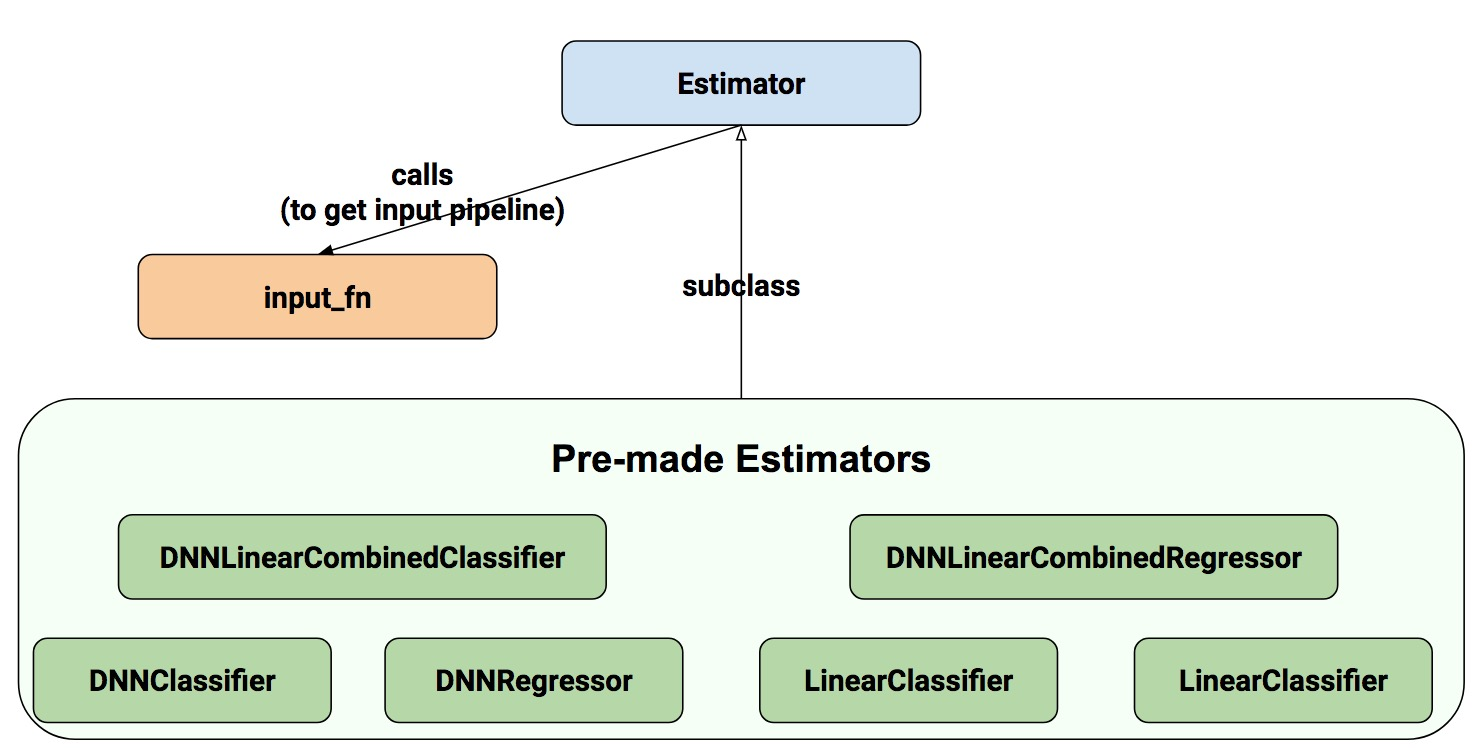[code lang=text]
# Create the feature_columns, which specifies the input to our model.
# All our input features are numeric, so use numeric_column for each one.
feature_columns = [tf.feature_column.numeric_column(k) for k in feature_names]

# Create a deep neural network regression classifier.
# Use the DNNClassifier pre-made estimator
classifier = tf.estimator.DNNClassifier(
feature_columns=feature_columns, # The input features to our model
hidden_units=[10, 10], # Two layers, each with 10 neurons
n_classes=3,
model_dir=PATH) # Path to where checkpoints etc are stored
[/code]

[code lang=text]
# Train our model, use the previously function my_input_fn
# Input to training is a file with training example
# Stop training after 8 iterations of train data (epochs)
classifier.train(
input_fn=lambda: my_input_fn(FILE_TRAIN, True, 8))
[/code]

FILE_TRAIN，训练数据文件。
True，告知估算器打乱数据。
8，告知估算器将数据集重复 8 次。

[code lang=text]
# Evaluate our model using the examples contained in FILE_TEST
# Return value will contain evaluation_metrics such as: loss & average_loss
evaluate_result = estimator.evaluate(
input_fn=lambda: my_input_fn(FILE_TEST, False, 4)
print("Evaluation results")
for key in evaluate_result:
print(" {}, was: {}".format(key, evaluate_result[key]))
[/code]

[code lang=text]
# Predict the type of some Iris flowers.
# Let's predict the examples in FILE_TEST, repeat only once.
predict_results = classifier.predict(
input_fn=lambda: my_input_fn(FILE_TEST, False, 1))
print("Predictions on test file")
for prediction in predict_results:
# Will print the predicted class, i.e: 0, 1, or 2 if the prediction
# is Iris Sentosa, Vericolor, Virginica, respectively.
print prediction["class_ids"]
[/code]

[code lang=text]
# Let create a memory dataset for prediction.
# We've taken the first 3 examples in FILE_TEST.
prediction_input = [[5.9, 3.0, 4.2, 1.5], # -> 1, Iris Versicolor
[6.9, 3.1, 5.4, 2.1], # -> 2, Iris Virginica
[5.1, 3.3, 1.7, 0.5]] # -> 0, Iris Sentosa
def new_input_fn():
def decode(x):
x = tf.split(x, 4) # Need to split into our 4 features
# When predicting, we don't need (or have) any labels
return dict(zip(feature_names, x)) # Then build a dict from them

# The from_tensor_slices function will use a memory structure as input
dataset = tf.contrib.data.Dataset.from_tensor_slices(prediction_input)
dataset = dataset.map(decode)
iterator = dataset.make_one_shot_iterator()
next_feature_batch = iterator.get_next()
return next_feature_batch, None # In prediction, we have no labels

# Predict all our prediction_input
predict_results = classifier.predict(input_fn=new_input_fn)

# Print results
print("Predictions on memory data")
for idx, prediction in enumerate(predict_results):
type = prediction["class_ids"] # Get the predicted class (index)
if type == 0:
print("I think: {}, is Iris Sentosa".format(prediction_input[idx]))
elif type == 1:
print("I think: {}, is Iris Versicolor".format(prediction_input[idx]))
else:
print("I think: {}, is Iris Virginica".format(prediction_input[idx])
[/code]

Dataset.from_tensor_slides() 面向可以装入内存的小数据集。按照与训练和评估时相同的方式使用 TextLineDataset 时，只要您的内存可以管理随机缓冲区和批次大小，您就可以处理任意大的文件。

[code lang=text]
# Replace PATH with the actual path passed as model_dir argument when the
# DNNRegressor estimator was created.
tensorboard –logdir=PATH
[/code]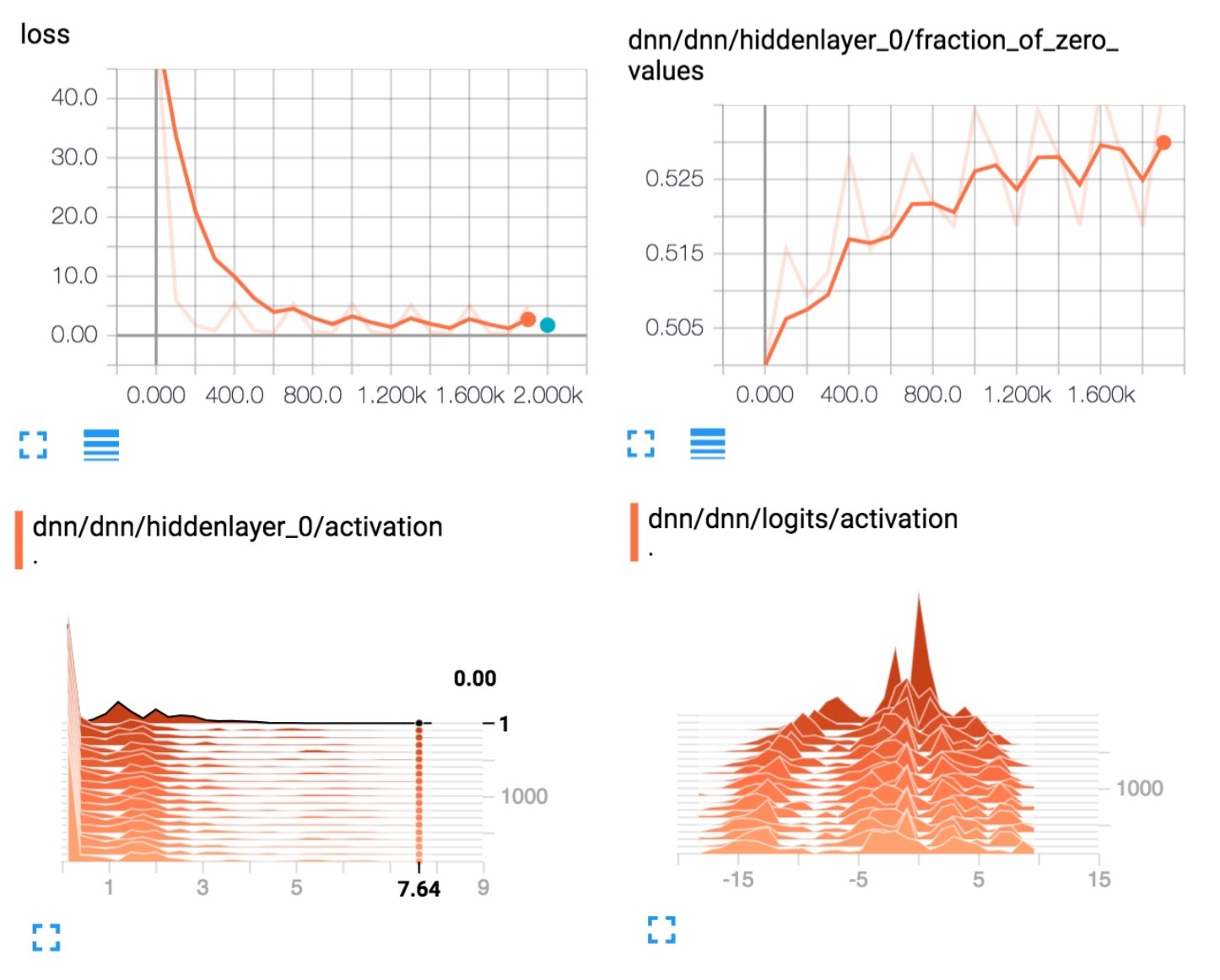Josh Gordon 有关这个问题非常不错的 Jupyter 笔记。使用这个笔记，您可以学习如何运行具有不同类型特征（输入）的更丰富示例。正如您从我们的模型中发现的一样，我们仅仅使用了数值特征。